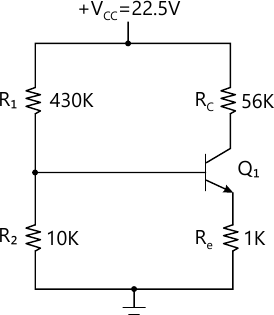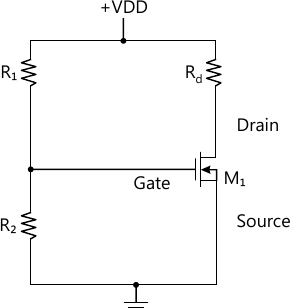MORE IN Electronic Devices & Circuits
SPPU Electronics and Telecom Engineering (Semester 3)
Electronic Devices & Circuits
December 2013
Total marks: --
Total time: --
INSTRUCTIONS
(1) Assume appropriate data and state your reasons
(2) Marks are given to the right of every question
(3) Draw neat diagrams wherever necessary

Solve any one question from Q1 and Q2
1 (a) For the circuit shown in the Figure No. 1, with transistor β=55, find the Q point and stability factor S.6 M
1 (b) Draw circuit diagram of a Darlington emitter follower. Explain why its input impedance is higher than that of a single-stage emitter follower. How to improve input impedance of Darlington emitter follower further high.
6 M

2 (a) Define Bias Stabilization and Compensation Techniques. Draw circuits which uses diode to compensate for changes in VBE and Ico.
6 M
2 (b) Determine Ai, Ais, Ri, Av, Avs, Ro for a Common Emitter BJT Amplifier having Rs=1 KΩ and RL=10 KΩ. The h-parameters for the BJT are, hie=1.1 KΩ, hre=2.5×10-4, hfe=50, hoe=25 μ. A/Volts. (Ignore effect of biasing resisters and Re is bypassed with high value capacitor).
6 M

Solve any one question from Q3 and Q4
3 (a) For BJT, at room temperature and Ic=1.3 mA, determine fβ and fT. Consider hfe=50, Ce=1 pF and Cc=0.2 pF.
6 M
3 (b) State effect of negative feedback on Input Impedance, Output Impedance, Gain, Bandwidth for Voltage Amplifier, Trans-conductance Amplifier, Trans-resistance Amplifier & Current Amplifier.
6 M

4 (a) Explain, how Step Response of an Amplifier can be used to determine its Bandwidth.
6 M
4 (b) Draw circuit diagram of transistorized Hartley Oscillator. Explain, how this circuit satisfies the minimum criteria to generate sustained oscillations. Calculate frequency of oscillations for the circuit if.
C=100 nF, L1=100 μH&L2=150μH.
6 M

Solve any one question from Q5 and Q6
5 (a) What is large signal amplifier? How it is different from small signal amplifier? Classify them on the basis of operating point position and compare their performance in terms of maximum conversion efficiency.
7 M
5 (b) A transistor amplifier supplies 5mW to a 100Ω load. Its DC collector current is 12rnA. If the second-harmonic distortion must stay within 10%, determine the peak collector current (with signal) allowed in the transistor.
6 M

6 (a) Draw circuit diagram of Class-B Push-Pull power amplifier using power BJTs. If BJTs in it are not perfectly matched, show output waveforms with sine wave input. Suggest changes in the circuit to eliminate any distortions that may occur in the output.
7 M
6 (b) A complementary - symmetry power amplifier has capacitive coupled load RL=8Ω supply voltage ±12V calculate,
i) Pac max.
ii) PD of each transistor
iii) Efficiency.
6 M

Solve any one question from Q7 and Q8
7 (a) The transistor in Figure 3 has parameters VTN=+2V and Kn=0.25 mA/V2. The circuit parameters are VDD=10V, R1=280 kΩ, R2=160 kΩ, and RD=10 kΩ. Find ID, VDS.6 M
7 (b) Explain the following non-ideal characteristics of MOSFET:
i) Breakdown effect,
ii) Body Effect,
iii) Subthreshold conduction,
iv) Temperature effect.
7 M

8 (a) Determine the small-signal voltage gain of a E-MOSFET Common Source circuit Assume circuit parameters: VGSQ=2.12 V, VDD=5V, and RD=2.5 kΩ. Assume transistor parameters: VTN=1V, K;=0.80 mA/V2, and λ=0.02V-1. Assume the transistor is biased in the saturation region.
6 M
Draw diagrams and state need of:
8 (b) (i) Constant Current Source Biasing Circuit for E-MOSFET.
4 M
8 (b) (ii) Bi-MOS Transistor.
3 M

More question papers from Electronic Devices & Circuits